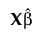Publication date: 04/12/2021

##Actual by Predicted Plot

The Actual by Predicted plot appears by default. It provides a visual assessment of model fit that reflects variation due to random effects. It plots the observed values of Y against the marginal predicted values of Y. These are the predicted values obtained if you select Save Columns > Prediction Formula.

Denote the linear mixed model by E[Y|γ] = Xβ + Zγ. Here β is the vector of fixed effect coefficients and γ is the vector of random effect coefficients. The marginal predictions are the predictions from the fixed effects part of the predictive model, given by.

Want more information? Have questions? Get answers in the JMP User Community (community.jmp.com).